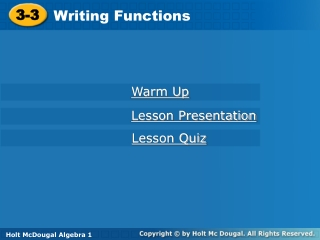DownloadDownload Presentation3-3

# 3-3

Download Presentation## 3-3

- - - - - - - - - - - - - - - - - - - - - - - - - - - E N D - - - - - - - - - - - - - - - - - - - - - - - - - - -
##### Presentation Transcript

1. 3-3 Writing Functions Holt Algebra 1 Warm Up Lesson Presentation Lesson Quiz Holt McDougal Algebra 1

2. Objectives Identify independent and dependent variables. Write an equation in function notation and evaluate a function for given input values.

3. Vocabulary independent variable dependent variable function rule function notation

4. Check It Out! Example 1 Determine a relationship between the x- and y-values. Write an equation. {(1, 3), (2, 6), (3, 9), (4, 12)} x 4 1 2 3 y 3 6 9 12 Step 1 List possible relationships between the first x- or y-values. 1  3 = 3 or 1 + 2 = 3

5. 2 • 3= 6 2 + 2 6 3 • 3 = 9 3 + 2 9 4 • 3 = 12 4 + 2 12 Check It Out! Example 1 Continued Step 2 Determine if one relationship works for the remaining values. The value of y is 3 times x. Step 3 Write an equation. y = 3x The value of y is 3 times x.

6. The equation in Example 1 describes a function because for each x-value (input), there is only one y-value (output).

7. The inputof a function is the independent variable. The output of a function is the dependent variable. The value of the dependent variable depends on, or is a function of, the value of the independent variable.

8. Helpful Hint There are several different ways to describe the variables of a function. Independent Variable Dependent Variable y-values x-values Domain Range Input Output x f(x)

9. Check It Out! Example 2b Identify the independent and dependent variable in the situation. Apples cost \$0.99 per pound. The cost of applesdepends on the number of pounds bought. Dependent variable: cost Independent variable: pounds

10. Example 3A: Writing Functions Identify the independent and dependent variables. Write an equation in function notation for the situation. A math tutor charges \$35 per hour. The fee a math tutor charges depends on number of hours. Dependent: fee Independent: hours Let h represent the number of hours of tutoring. The function for the amount a math tutor charges isf(h) = 35h.

11. Example 3B: Writing Functions Identify the independent and dependent variables. Write an equation in function notation for the situation. A fitness center charges a \$100 initiation fee plus \$40 per month. The total cost depends on the number of months, plus \$100. Dependent: total cost Independent: number of months Let mrepresent the number of months The function for the amount the fitness center charges is f(m) = 40m + 100.

12. Check It Out! Example 3b Identify the independent and dependent variables. Write an equation in function notation for the situation. An amusement park charges a \$6.00 parking fee plus \$29.99 per person. The total cost depends on the number of persons in the car, plus \$6. Dependent: total cost Independent: number of persons in the car Let xrepresent the number of persons in the car. The function for the total park cost is f(x) = 29.99x+ 6.

13. Example 4A: Evaluating Functions Evaluate the function for the given input values. For f(x) = 3x + 2, find f(x) when x = 7 and when x = –4. f(x) = 3(x) + 2 f(x) = 3(x) + 2 Substitute 7 for x. Substitute –4 for x. f(–4) = 3(–4) + 2 f(7) = 3(7) + 2 Simplify. = –12 + 2 = 21 + 2 Simplify. = 23 = –10

14. Example 4B: Evaluating Functions Evaluate the function for the given input values. For g(t) = 1.5t – 5, find g(t) when t = 6 and when t = –2. g(t) = 1.5t– 5 g(t) = 1.5t– 5 g(6) = 1.5(6) – 5 g(–2) = 1.5(–2) – 5 = –3– 5 = 9– 5 = 4 = –8

15. For , find h(r) when r = 600 and when r = –12. Example 4C: Evaluating Functions Evaluate the function for the given input values. = 202 = –2

16. Check It Out! Example 4a Evaluate the function for the given input values. For h(c) = 2c – 1, find h(c) when c = 1 and when c = –3. h(c) = 2c– 1 h(c) = 2c– 1 h(1) = 2(1) – 1 h(–3) = 2(–3) – 1 = 2– 1 = –6– 1 = 1 = –7

17. Lesson Quiz: Part I Identify the independent and dependent variables. Write an equation in function notation for each situation. 1. A buffet charges \$8.95 per person. independent: number of people dependent: cost f(p) = 8.95p 2. A moving company charges \$130 for weekly truck rental plus \$1.50 per mile. independent: miles dependent: cost f(m) = 130 + 1.50m

18. Lesson Quiz: Part II Evaluate each function for the given input values. 3. For g(t) = , find g(t) when t = 20 and when t = –12. g(20) = 2 g(–12) = –6 4. For f(x) = 6x – 1, find f(x) when x = 3.5 and when x = –5. f(3.5) = 20 f(–5) = –31

19. Lesson Quiz: Part III Write a function to describe the situation. Find the reasonable domain and range for the function. 5. A theater can be rented for exactly 2, 3, or 4 hours. The cost is a \$100 deposit plus \$200 per hour. f(h) = 200h + 100 Domain: {2, 3, 4} Range: {\$500, \$700, \$900}